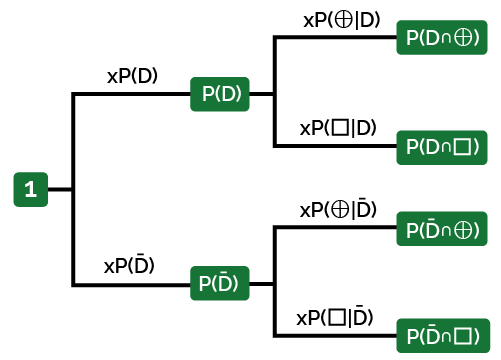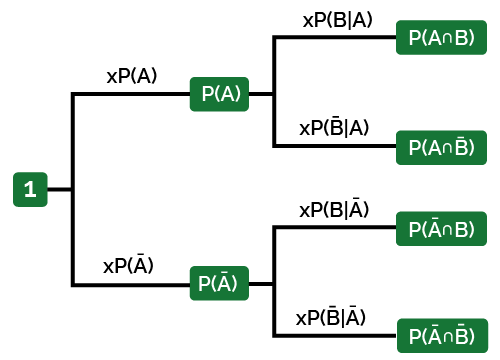# Conditional Probability Calculator

Created by Tibor Pal, PhD candidate
Reviewed by Dominik Czernia, PhD candidate and Jack Bowater
Last updated: Feb 21, 2022

The conditional probability calculator helps you to determine the probability of an event occurring, provided it is conditional on another event.

Read further and we explain:

• What conditional probability is;
• How to calculate conditional probability; and
• In addition, we show you a real-life conditional probability example where you can also learn how to find it in practice.

If you would like to discover the connection between conditional probability and Bayes' theorem, you may check our Bayes' theorem calculator.

## Conditional probability definition - What is conditional probability?

In probability theory, conditional probability quantifies the probability (or likelihood) of an event occurring given that another event has already occurred. This requires that probability of the second event occurring is affected by the first event happening. It is computed by multiplying the probability of the first event by the updated probability of the second, or conditional, event.

Which situations involve conditional probability? Keep reading the article to find it out!

## How do I calculate conditional probability?

You need the take the following steps to compute the conditional probability of P(A|B):

1. Determine the total probability of a given final event, B:

P(B) = P(A∩B) + P(Ā∩B) = P(A) * P(B|A) + P(Ā) * P(B|Ā)

2. Compute the probability of that event:

P(A∩B) = P(A) * P(B|A)

3. Divide the two numbers:

P(A|B) = P(A∩B) / P(B)

## Conditional probability examples and solutions

Let's consider a real-life example to demonstrate how to find conditional probability and show the relevance of conditional probability.

Assume that in a population, 5 percent are affected by a disease (denoted by $D$). A test is available to check if a given person is infected.

The test's sensitivity (also known as the true positive rate) is 91%, meaning that if the person is infected, the probability for a negative test result (denoted by $\Box$) is 0.09. On the other hand, the test's specificity (or true negative rate) is 95%, implying that the probability of having a positive test result (assigned by $\oplus$) when the person doesn't have the disease is 0.05.

Now, let's consider a random person from the population taking the test and receiving a positive result. How do you find the conditional probability that the person really does have the disease? We formulate it as $P(D \cap \oplus)$, that you read as the conditional probability of being infected given that the person has a positive test result.

We can summarize the above problem with the following conditional probability tree diagram:Translating into mathematical formulas, we can obtain the following equations explaining how to find conditional probability:

$\scriptsize P(D) = 0.05 \\ P(\bar D) = 1 - 0.05 = 0.95\\ P(\Box \mid D)= 0.09 \\ P(\oplus \mid D) = 1 - 0.09 = 0.91 \\ P(\oplus\mid \bar D) = 0.05\\ P(\Box\mid \bar D) = 1 - 0.05 = 0.95 \\$

So, how to solve conditional probability in this situation?

You need to take the following steps:

1. Compute the total probability of having a positive result:
$\scriptsize P(\oplus) = P(D \cap \oplus)+P(\bar D \cap \oplus)=\\ = P(D) \times P(\oplus \mid D) + P(\bar D) \times P(\oplus\mid \bar D) =\\ = 0.91 \times 0.05 + 0.05 \times 0.95 = 0.093$
1. Compute the probability of an event when the random person is infected and the test result is positive. You can use the conditional probability definition:
$\scriptsize P(D \cap \oplus) =P(D) \times P(\oplus \mid D) = \\ = 0.91 \times 0.05 = 0.0455$
1. Divide the two numbers, taking into account the conditional probability rules:
$\scriptsize P(D \mid \oplus) = \frac {P(D \cap \oplus)}{P(\oplus)} = \frac {0.0455}{0.093} = 0.4892\\$

Thus, the conditional probability that a random person is infected that has a positive test result is 0.4892, which is almost fifty percent - nearly analogical to tossing a fair coin. You can try to verify the result with our conditional probability calculator if you want.

Note, that the above-described situation may represent the early stages of the COVID pandemic. Even though only a tiny portion of the population has been infected (thus having antibodies), some countries strongly supported wide-range testing based on antibodies with nearly the same parameters. Therefore, it is particularly important to consider the entire clinical state of a patient with signs and symptom before taking the test, as they may present a positive result without being infected. Taking these steps increases the accuracy of the test result.

## FAQ

### Which situations involve conditional probability?

There are many real-life situations where conditional probability matters. For example, the probability of winning the second round of a game given that you won the first round. Or the probability that it will rain given that it is cloudy.

### What is the conditional probability rule?

Conditional probability measures the chances that an event occurs, given that another event has also occurred. Typically, it is stated as P(B|A) (read as the probability of B given A), where the probability of B depends on the probability of A's occurrence.

### Can the conditional probability be zero?

Yes. If the probability of a given final event is zero, the conditional probability of a previous event given that final event is zero.

Tibor Pal, PhD candidate
Event A
P(A)
P(B | A)
P(B̅ | A)
P(A ∩ B)
P(A ∩ B̅)
Event Ā
P(Ā)
P(B | Ā)
P(B̅ | Ā)
P(Ā ∩ B)
P(Ā ∩ B̅)
Total probabilities
P(B)
P(B̅)
Conditional events
P(A | B)
P(A | B̅)
P(Ā | B)
P(Ā | B̅)
Conditional probability tree diagramPeople also viewed…

### 6 sided dice probability

Use 6 sided dice probability calculator to find the likelihood of rolling numerous numbers sets with standard cubic dice.

### Post test probability

The post-test probability calculator allows you to calculate pre-test probability, likelihood ratios, and finally, the post-test probability itself.

### Secretary problem (Valentine's day)

Use the dating theory calculator to enhance your chances of picking the best lifetime partner.

### Snowman

The perfect snowman calculator uses math & science rules to help you design the snowman of your dreams!